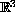2002-Fez conference on Partial Differental Equations,
Electron. J. Diff. Eqns., Conf. 09, 2002, pp. 65-76.

### Stationary solutions for a Schrodinger-Poisson system inKhalid Benmlih

Abstract:
Under appropriate, almost optimal, assumptions on the data we prove existence of standing wave solutions for a nonlinear Schrodinger equation in the entire spacewhen the real electric potential satisfies a linear Poisson equation.

Published December 28, 2002.
Subject classfications: 35J50, 35Q40.
Key words: Poisson equation, standing wave solutions, variational methods.

Show me the PDF file (213K), TEX file, and other files for this article.Khalid Benmlih Department of Economic Sciences, University of Fez P.O. Box 42A, Fez, Morocco. E-mail: kbenmlih@hotmail.com